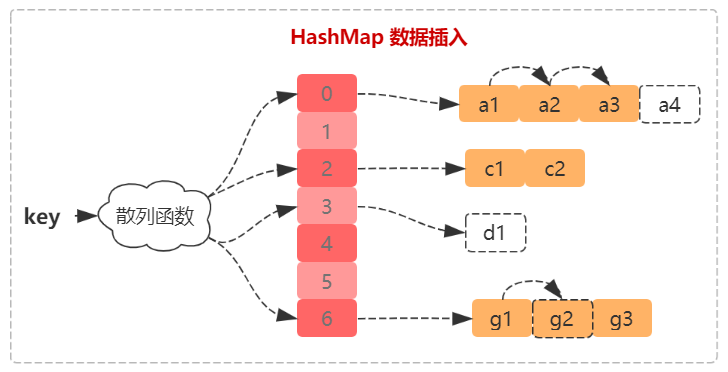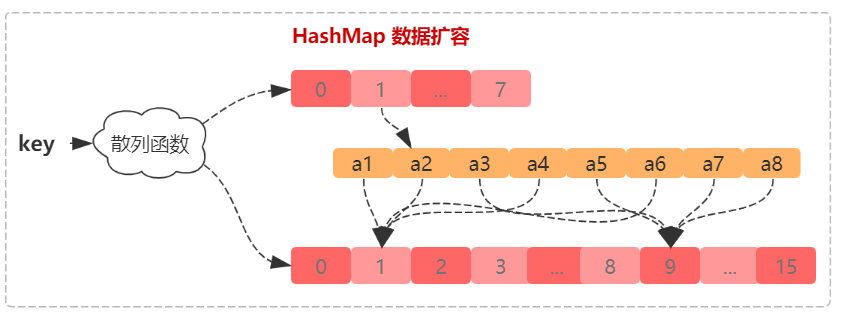# Java 数据结构 - HashMap 源码解读：如何设计工业级的散列表

Java 数据结构 - 散列表原理一文中，提到评价一个散列表的标准有三个：散列函数、散列冲突、加载因子（动态扩容）三个指标。那像 HashMap 这样工业级的散列表应该具有哪些特性？

• 支持快速的查询、插入、删除操作，时间复杂度为 O(1)；
• 内存占用合理，不能浪费过多的内存空间；
• 性能稳定，极端情况下，散列表的性能也不会退化到 O(n)，以至于无法接受。

## 1. HashMap 三大指标分析

### 1.1 如何设计散列函数

static final int hash(Object key) {
int h;
return (key == null) ? 0 : (h = key.hashCode()) ^ (h >>> 16);
}


1. 将 hashCode 的高 16 位和低 16 位进行异或运算，进一步保证哈希函数的随机性和均匀性。
2. 散列表的长度必须是 2^n，直接使用位运算进行求余 i = (n - 1) & hash(kye)

public int hashCode() {
int h = hash;
if (h == 0 && value.length > 0) {
char val[] = value;
for (int i = 0; i < value.length; i++) {
h = 31 * h + val[i];
}
hash = h;
}
return h;
}


### 1.2 如何选择散列冲突解决方法

• 线性探测法：数据量比较小、装载因子小。当装载因子 loadfactor 接近 1 时，散列冲突会非常严重。
• 拉链法：存储大对象、大数据量。对装载因子较大的容忍度高。

• 当链表长度太长（默认超过 8）时，链表就转换为红黑树。我们可以利用红黑树快速增删改查的特点，提高 HashMap 的性能。

• 当红黑树结点个数少于 8 个的时候，又会将红黑树转化为链表。因为在数据量较小的情况下，红黑树要维护平衡，比起链表来，性能上的优势并不明显。但 HashMap 也不是直接使用链表，当链表的长度大于 8 时会转换会红黑树。

### 1.3 装载因子多大合适：什么时候触发动态扩容

散列表的装载因子 = 表中的元素个数 / 散列表的长度


HashMap 中装载因子动态扩容问题：

• 装载因子和动态扩容。最大装载因子默认是 loadFactor = 0.75，当 HashMap 中元素个数超过 0.75 * capacity（capacity 表示散列表的容量）的时候，就会启动扩容，每次扩容都会扩容为原来的两倍大小。

• 初始大小。HashMap 默认的初始大小是 16。如果事先知道大概的数据量有多大，可以通过修改默认初始大小，减少动态扩容的次数，这样会大大提高 HashMap 的性能。

## 2. 源码解读

### 2.1 重要属性

（1）扩容相关属性

static final float DEFAULT_LOAD_FACTOR = 0.75f;
static final int DEFAULT_INITIAL_CAPACITY = 1 << 4;
static final int MAXIMUM_CAPACITY = 1 << 30;

transient Node<K,V>[] table;  // 表示hash数组，数组长度必须为 2^n
transient int size;           // 表示当前表中元素的个数

// 当threshold>size时扩容。threshold = table.length * loadFactor
int threshold;


（2）红黑树相关属性

static final int TREEIFY_THRESHOLD = 8;    // 链表转红黑树的阀值
static final int UNTREEIFY_THRESHOLD = 6;  // 红黑树转链表的阀值
// 如果HashMap的容量小于64先启动扩容，只有容量大于64才会转红黑树
static final int MIN_TREEIFY_CAPACITY = 64;


### 2.2 插入HashMap 中插入 key 时，有以下几种可能：

1. hash(key) 对应的数组没有元素。如插入 key = d1 的元素。
2. 有元素已经存在，并且是红黑树。按红黑树处理即可，红黑树不是本文分析的重点。
3. 有元素已经存在，并且结构是链表。这时有两种情况：
• key 已经存在，需要替换这个 key。如插入 key = g2 的元素。
• key 不存在，直接插入到链表尾。这时还需要判断链表的长度是否大于 8，否则还需要将链表转红黑树。如插入 key = a4 的元素。
/**
* @param onlyIfAbsent 只有元素不存在时才插入
*/
final V putVal(int hash, K key, V value, boolean onlyIfAbsent, boolean evict) {
Node<K,V>[] tab; Node<K,V> p; int n, i;
// 1. 初始化数组
if ((tab = table) == null || (n = tab.length) == 0)
n = (tab = resize()).length;
// 2. 有空闲位置，直接存储即可
if ((p = tab[i = (n - 1) & hash]) == null)
tab[i] = newNode(hash, key, value, null);
// 3. 没有空闲位置，二种情况：可能是hash碰撞，也可能是该key对应的元素已经存在
else {
Node<K,V> e; K k;
// 3.1 元素已经存在，e变量保存旧值
if (p.hash == hash &&
((k = p.key) == key || (key != null && key.equals(k))))
e = p;
// 3.2 红黑树，先不管
else if (p instanceof TreeNode)
e = ((TreeNode<K,V>)p).putTreeVal(this, tab, hash, key, value);
// 3.3 遍历链表，如果key已经存在则保存到e中，如果不存在则直接追加到链表尾
else {
for (int binCount = 0; ; ++binCount) {
// 3.3.1 key不存在，直接追加不链表尾
if ((e = p.next) == null) {
p.next = newNode(hash, key, value, null);
// 判断链表是否要转红黑树
if (binCount >= TREEIFY_THRESHOLD - 1) // -1 for 1st
treeifyBin(tab, hash);
break;
}
// 3.3.2 key存在，保存到到e中
if (e.hash == hash &&
((k = e.key) == key || (key != null && key.equals(k))))
break;
p = e;
}
}
// 3.4 key已经存在，判断是否替换旧值
if (e != null) { // existing mapping for key
V oldValue = e.value;
if (!onlyIfAbsent || oldValue == null)
e.value = value;
afterNodeAccess(e);
return oldValue;
}
}
// 4. 判断是否需要扩容
++modCount;
if (++size > threshold)
resize();
afterNodeInsertion(evict);
return null;
}


### 2.3 查找

final Node<K,V> getNode(int hash, Object key) {
Node<K,V>[] tab; Node<K,V> first, e; int n; K k;
// hash(key) 对应的数组中存在数据，需要进一步查找对应的 key
if ((tab = table) != null && (n = tab.length) > 0 &&
(first = tab[(n - 1) & hash]) != null) {
// 1. 判断链表的第一个结点是不是指定的 key
if (first.hash == hash && // always check first node
((k = first.key) == key || (key != null && key.equals(k))))
return first;
// 2. 链表或红黑树递归查找 key
if ((e = first.next) != null) {
if (first instanceof TreeNode)
return ((TreeNode<K,V>)first).getTreeNode(hash, key);
do {
if (e.hash == hash &&
((k = e.key) == key || (key != null && key.equals(k))))
return e;
} while ((e = e.next) != null);
}
}
return null;
}


### 2.5 扩容

HashMap 扩容首先要确定扩容后的数组长度，再进行数据搬移。

HashMap 默认按原数组的 2 倍扩容。如果数组未初始化，则需要先进行初始化。初始化分如果没有指定数组初始化容量，按默认容量 16 进行初始化。如果指定了初始化容器 threshold（一定是 2n)，则按照 threshold 初始化，并根据加载因子重新计算扩容阀值 threshold = newCap * loadFactorfinal Node<K,V>[] resize() {
// 1. 确定数组扩容后的长度，默认的按2倍扩容
Node<K,V>[] oldTab = table;
int oldCap = (oldTab == null) ? 0 : oldTab.length;
int oldThr = threshold;
int newCap, newThr = 0;
// 1.1 如果数组已经初始化，按原数组的2倍大小扩容
if (oldCap > 0) {
if (oldCap >= MAXIMUM_CAPACITY) {
threshold = Integer.MAX_VALUE;
return oldTab;
} else if ((newCap = oldCap << 1) < MAXIMUM_CAPACITY &&
oldCap >= DEFAULT_INITIAL_CAPACITY)
newThr = oldThr << 1; // double threshold
// 1.2 原数组未初始化，但设置初始化长度，按初始化长度进行初始化
} else if (oldThr > 0) // initial capacity was placed in threshold
newCap = oldThr;
// 1.3 原数组未初始化，按默认大小初始化数组
else {               // zero initial threshold signifies using defaults
newCap = DEFAULT_INITIAL_CAPACITY;
}
if (newThr == 0) {
float ft = (float)newCap * loadFactor;
newThr = (newCap < MAXIMUM_CAPACITY && ft < (float)MAXIMUM_CAPACITY ?
(int)ft : Integer.MAX_VALUE);
}
threshold = newThr;

// 2. 数据搬移
Node<K,V>[] newTab = (Node<K,V>[])new Node[newCap];
table = newTab;
if (oldTab != null) {
for (int j = 0; j < oldCap; ++j) {
Node<K,V> e;
if ((e = oldTab[j]) != null) {
oldTab[j] = null;
if (e.next == null)
newTab[e.hash & (newCap - 1)] = e;
else if (e instanceof TreeNode)
((TreeNode<K,V>)e).split(this, newTab, j, oldCap);
else { // preserve order
// 链表结构的数据搬移
Node<K,V> loHead = null, loTail = null;
Node<K,V> hiHead = null, hiTail = null;
Node<K,V> next;
do {
next = e.next;
if ((e.hash & oldCap) == 0) {
if (loTail == null)
else
loTail.next = e;
loTail = e;
} else {
if (hiTail == null)
else
hiTail.next = e;
hiTail = e;
}
} while ((e = next) != null);
if (loTail != null) {
loTail.next = null;
}
if (hiTail != null) {
hiTail.next = null;
}
}
}
}
}
return newTab;
}


posted on 2020-03-10 13:23  binarylei  阅读(142)  评论(0编辑  收藏

• 随笔 - 425
• 文章 - 0
• 评论 - 15
• 引用 - 0